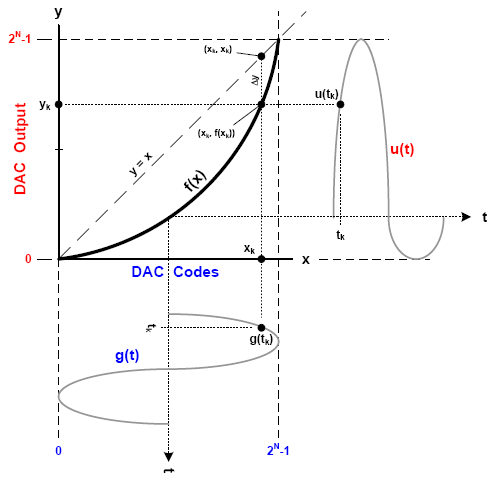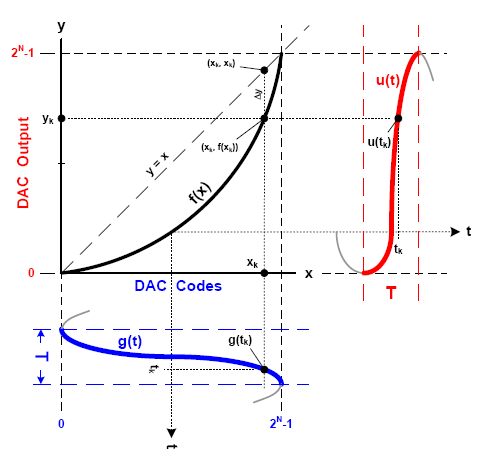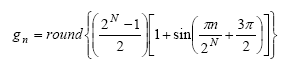# Reconstruct a DAC Transfer Function from its Harmonic Spectral Content

All DACs exhibit some degree of harmonic distortion, which is a measure of how well the DAC reproduces a perfect sinusoid at its output when its input is driven with a numeric sequence representing an ideal uniformly sampled sinusoid. The output spectrum will contain harmonic content due to the DAC’s non-ideal transient and static behavior. The transient output characteristics of the DAC include slew rate limitations, asymmetrical rise and fall times, and finite settling time. The static characteristics relate to how the transfer function deviates from a straight line. This article focuses on the static behavior, and defines a method for deriving the DAC transfer function from the harmonic content observed in the output spectrum. The analysis assumes that the transfer function, rather than the transient output characteristics, is the dominant source of the observed harmonic distortion. This assumption is valid at low frequencies.

### The DAC Transfer Function

Figure 1 shows an ideal DAC transfer function, which is the diagonal straight line, y=mx+b. The digital inputs reside on the x-axis and the analog output resides on the y-axis.Figure 1. An ideal DAC transfer function.

The range of interest on the x-axis is from the smallest code on the left (A) to the largest code toward the right (B). The range of interest on the y-axis is from the lowest output value at the bottom (C) to the largest output value near the top (D). The equations defining the slope (m) and y-intercept (b) of the ideal transfer function are shown in terms of the boundary values, A, B, C, and D. The signal g(t) represents an undistorted sine wave consisting of digital inputs in the range of A and B with time progressing downward. The signal u(t) represents the analog output, which spans values from C to D with time progressing to the right.

The output signal is a reflection of the input signal via the transfer function. Notice that the output signal is the result of linking each point on g(t) to its associated point on u(t). Figure 1 shows an example of the transfer operation at the specific time instant, t=tk, which identifies point g(tk) on the input signal. The transfer function, in turn, links g(tk) to the corresponding point, u(tk), on the output signal. With an ideal linear transfer function, u(t) will be a scaled version of g(t). Note that g(tk) corresponds to point xk on the x-axis, which reflects, via the transfer function, to point yk on the y-axis. Prior knowledge of the coupled sets of points (g(tn),u(tn)) makes it possible to identify the associated points (xn,yn) on the transfer function. Thus, the relationship between points on the input signal, g(t), and points on the output signal, u(t), completely defines the transfer function.

For an N-bit DAC, boundary values A and B take on specific values; namely, A = 0 and B = 2N–1. Boundary values C and D, on the other hand, are conveniently assigned as C = A and D = B. This assignment implies the scale and offset of the actual DAC output signal so that its peak-to-peak span is from 0 to 2N–1. Using these values of A, B, C, and D, the ideal transfer function simplifies to y = x because the slope and intercept become m = 1 and b = 0.

Up to this point, the focus has been on an ideal DAC transfer function, but we now have the tools to deal with a distorted DAC transfer function, f(x), as shown in Figure 2. The main feature to note is that the transfer function is no longer the straight line, y = x, but the shaped function, f(x), arbitrarily shown here as a smooth arc. Of equal importance is the effect that f(x) has on the output function, u(t). The ideal input, g(t), reflects off the transfer function, f(x), to produce the distorted output, u(t). The transfer function shown is highly exaggerated compared to that of any off-the-shelf DAC, with the dramatic arc shown for illustrative purposes only. The transfer function of modern DACs barely deviates from the ideal straight line, but even the slightest deviation causes harmonic spurs to appear in the output spectrum.Figure 2. A distorted DAC transfer function.

Successful reconstruction of the DAC transfer function relies on the ability to determine each point, (xk,f(xk)), from knowledge of g(t) and u(t). This is a two-step process: first drive the DAC input with a numeric sequence representing a perfectly sampled sinusoid, measure the DAC output with a spectrum analyzer, and record the magnitudes of the fundamental signal and as many harmonics as possible; then convert the measured harmonic magnitudes into a transfer function with a specific shape. If done properly, simulating u(t) by passing g(t) through f(x) will yield the same harmonic distortion values as those measured.

### Step I: Measure the DAC Harmonics

The first step requires an input sequence that represents one cycle of a perfect sinusoid sampled at uniformly spaced time intervals. The goal is to reconstruct the DAC transfer function, so the input signal must contain at least one occurrence of every DAC code from 0 to 2N–1. The input sequence requires more than 2N samples to exercise every DAC code with equally spaced samples, and it actually takes at least 2N+3 samples to guarantee that every code is hit. The following formula produces a perfect sinusoidal sequence of 2K DAC codes with K ≥ N+3. The function, round{x}, rounds x to the nearest integer.where n=0,1,2,3, ... 2K–1

This equation assumes that the DAC decodes digital input words in straight binary format, as unsigned integers from 0 to 2N–1. For offset binary or two’s-complement DACs, gn must be adjusted to indicate negative values.

The numeric sequence (gn) is repetitively delivered to the DAC at sample rate fs, so the DAC output spectrum contains the fundamental signal at frequency f0=fs/2K. The harmonics appear at 2f0, 3f0, 4f0, and other integer multiples of f0. The magnitude of these harmonics is subject to a sin(x)/x response due to the sampled nature of the DAC output spectrum. Because f0 is such a small fraction of fs, however, the sin(x)/x response is virtually flat and can be ignored. With an 8-bit DAC, for example, K ≥ 11, and f0 ≤ fs/2048, so the sin(x)/x will be no more than 0.39% (0.034 dB) out to the 100th harmonic.

Accurately reconstructing the transfer function, f(x), requires recording the magnitude of as many harmonics as possible based on a set of harmonic numbers (h). These integers start with h = 1 (the fundamental frequency) and end with h=H, where H is the highest harmonic number for which a measured magnitude is taken. For example, for measurements taken out to the 10th harmonic, H = 10 and the set of harmonic numbers is h={1, 2, 3, .. 10}.

Next, associate the magnitude (M) of each measured harmonic with its harmonic number. For example, M1 is the magnitude of the 1st harmonic (the fundamental), M2 is the magnitude of the 2nd harmonic, and so on through MH. Harmonic magnitudes are usually measured in decibels relative to the magnitude of the fundamental (dBc). Convert dBc to linear units by:

where D is the measured harmonic magnitude in dBc. If, for example, the magnitude of the 3rd harmonic is –40 dBc, then the linear magnitude is M3 = 10–40/20, or 0.01. M1 always equals 1, because the magnitude of the fundamental is 0 dBc, by definition.

### Step II: Reconstructing the DAC Transfer Function

The second step of the process involves relating the measured harmonics to the transfer function. The points on f(x) depend on the relationship between corresponding points on g(t) and u(t), so the harmonic magnitudes in the frequency domain must first be converted to a time domain representation. Note that g(t) consists of DAC codes that have a one-to-one correspondence with the time points associated with the sinusoidal form of g(t). The DAC codes comprising g(t) thus relate to the time domain. Furthermore, since u(t) relates to g(t) via f(x), and g(t) is a time domain function, then u(t) must be expressed as a time domain function, as well. This allows each time point, tk, in g(t) to be linked to its associated time point in u(t), which is necessary in order to determine f(x) from g(t) and u(t).

Converting the harmonic magnitudes to a time domain representation is challenging, because f(x) must relate unambiguously to each possible DAC code (0 to 2N–1) in g(t). Since g(t) is a perfect sinusoid, the only way to ensure uniqueness is to limit the range to where the sinusoid is monotonically increasing, as shown in the highlighted section of Figure 3. Without such a limitation, a single point on f(x) could map to two points on g(t) and cause ambiguity.

To demonstrate such ambiguity, imagine sliding region T downward. The point (xk, f(xk)) on f(x) could now be associated with two points on g(t), an unacceptable occurrence. By limiting the range of T to that shown, ambiguity cannot exist. Because g(t) is a sinusoid, the desired range of T corresponds to ½ cycle with an initial phase offset of 3π/2 radians.Figure 3. Relationship between f(x) and g(t).

The fact that g(t) is bounded by T implies a similar bound on u(t). Hence, the conversion of the recorded harmonic magnitudes to the time domain must ensure confinement of u(t) to the same range of T as g(t), as shown in Figure 4.Figure 4. Time domain range of g(t) and u(t).

Note that the actual time span T is irrelevant, because f(x) serves only to translate between the amplitudes of g(t) and u(t). To simplify the analysis, normalize the fundamental frequency (f0) to 1. The frequency of the 2nd harmonic is therefore 2, the frequency of the 3rd harmonic is 3, and so on. As a result, the harmonic frequency is identical to the harmonic number (h): fh=h. This convenient relationship simplifies the mathematics used to create u(t) from the harmonic measurements, Mh.

The general time domain representation of a sine wave is:where β is peak amplitude; θ is initial phase offset

The time domain representation of each harmonic, uh(t), can be realized by substituting h for f and Mh for β. Recall, however, that g(t) is offset by 3π/2 radians. Furthermore, the link between g(t) and u(t) via f(x) implies that g(t) and u(t) be aligned in phase. Substituting 3π/2 for θ provides the required alignment. In the following equation, note that 0 ≤ t < 1 and π replaces 2π to limit the fundamental to a half cycle as indicated by interval T:

With knowledge of the time domain representation of each harmonic, uh(t), it is possible to reconstruct the composite output, u(t), as the sum of the fundamental and harmonic signals:

Recall that the goal is to reconstruct the DAC transfer function, f(x), by relating g(t) and u(t). Furthermore, g(t) must consist of exactly 2N samples in order maintain a one-to-one correspondence with the points on f(x). Hence, calculate the samples of g(t) as:(n=0,1,2,3 .. 2N–1)

Since g(t) consists of 2N samples, it seems reasonable to reconstruct f(x) from a u(t) sample set consisting of 2N samples. It turns out, however, that at least 2N+3 samples are necessary to provide suitable accuracy for small values of Mh. With this in mind, calculate each sample of u(t) as follows:(n=0,1,2,3 .. 2N+3–1)

Note that this results in u(t) containing more samples than g(t). This complicates the mapping of u(t) and g(t) onto f(x), because multiple samples of u(t) can correspond to a single point on f(x) and g(t). Hence, specific groups of samples must be averaged in order to provide a reasonable mapping onto f(x). The following pseudo-code demonstrates the required mapping assuming an N-bit DAC, 2N points of g(t), and 2N+3 points of u(t). The array, DacXfr, consists of 2N elements, which are initially zero. After executing the code, the elements of the DacXfr array contain the normalized DAC transfer function.

n = 0
FOR i = 0 TO 2N–1
AvgCnt = 0
WHILE i = g[n]
AvgCnt = AvgCnt + 1
DacXfr[i] = DacXfr[i] + u[n]
n = n + 1
IF n >= 2N+3
EXIT WHILE
END IF
END WHILE
IF AvgCnt = 0
EXIT (fail because array, g[ ], is missing a DAC code)
END IF
DacXfr[i] = (DacXfr[i]/AvgCnt)/2N
END FOR

### Validation

To validate the methods described in this article, a spectrum analyzer was used to measure the output of a 14-bit DAC driven by an input sequence representing a perfect sinusoid. The magnitudes of the first fourteen harmonics (number 2 through 15, in units of dBc) were recorded and the values were used to reconstruct the DAC transfer function, f(x). Next, a simulation produced an output sequence, u(t), by passing an ideal sinusoidal input sequence, g(t), through the reconstructed DAC transfer function, f(x). An FFT converted u(t) to its frequency domain equivalent, U(ω). The harmonic magnitudes were extracted from U(ω) and compared to the spectrum analyzer measurements, as shown in the tabulated results of Table 1. Note that the largest error, associated with the 7th harmonic, is only 0.065 dB.

Table 1
 Harmonic Number Measured Magnitude (dBc) Simulated magnitude (dBc) Deviation (dB) 1 0.00 0.00 0.000 2 -75.1 -75.100 0.000 3 -74.5 -74.502 -0.002 4 -90.5 -90.501 -0.001 5 -86.5 -86.498 0.002 6 -92.0 -91.999 0.001 7 -95.5 -95.565 -0.065 8 -93.8 -93.801 -0.001 9 -97.2 -97.187 0.013 10 -89.6 -89.599 0.001 11 -94.2 -94.204 -0.004 12 -98.8 -98.802 -0.002 13 -95.6 -95.649 -0.049 14 -99.3 -99.298 0.002 15 -91.1 -91.080 0.020

Due to the scale, a plot of the reconstructed transfer function appears as a straight line (y = x). In fact, the transfer function actually deviates from y = x enough to produce the harmonic content shown in Table 1. It helps to plot only the deviation of the transfer function from the ideal straight line, as shown in Figure 5. The vertical axis is in units of LSBs.Figure 5. Residual error of DAC transfer function.